﻿ Ferromagnetism by de Broglie waves

# Ferromagnet --- truth

## Unreal electron spin causes ferromagnet ?

### [ The present physics claims "spin alignment" causes ferromagnet. ]

(Fig.1)  But electron "spinning" speed is much faster than light, so unreal !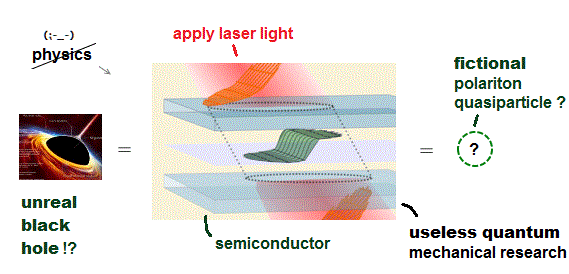It is known that certatin materials such as iron form permanent magnets, or are attracted to magnets, which is called ferromagnetism.

The present quantum mechanics claims when electron spins are aligned parallel, it causes ferromagnetism in iron.

But an electron is so tiny and point-like that its spinning speed must be much faster than light ( > c ) to cause its magnetic field.  So this "spin" model is unrealistic.

## Electron spin in metal is unreal.

### [ Spin is too weak to cause ferromagnet. ]

(Fig.2)  ↓ Spin model is broken at room temperature !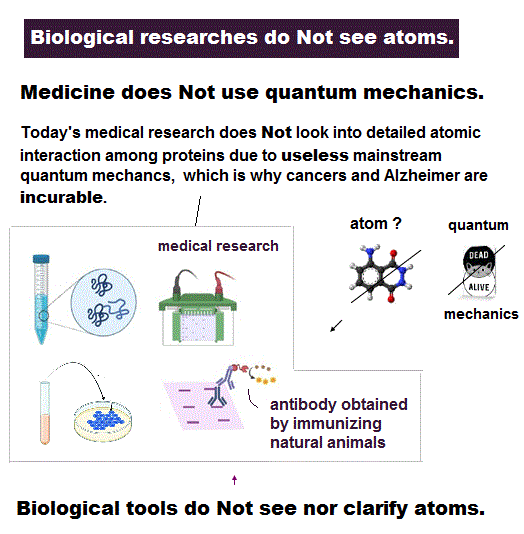Quantum mechanics claims that (anti)ferromagnetism is caused by electron spin.  But this is a big lie.

The magnetic energy by electron spin is too weak to cause ferromagnet.
Spin-spin interaction is easily broken at low temperature ( ~ 0.3 K ).

But actual iron can keep ferromagnetic at 1043 K ( this p.7 ).

In fact, electron's spin disagrees with many experimental results !
"Spin" is used only as a "mark" with No physical meaning.

So we must give up contradictory spin model to investigate further mechanism !

It's natural to think that ferromagnet is maintained stably by Coulomb interaction among electrons' orbits ( not spin ! )

## Ferromagnet has nothing to do with spin.

### [ Spin magnetic moment is too weak to explain ferromagnetism. ]

(Fig.3)  Spin magnet is too weak to explain ferromagnet.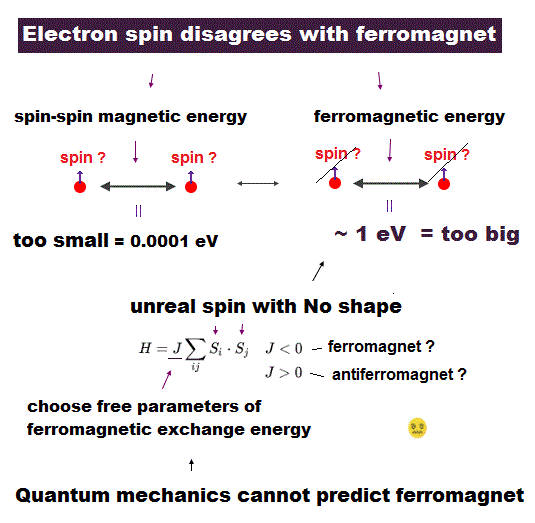You may think Spintronics and excitonics are useful (← ? ) for your career.

But almost nobody knows electron spin lacks reality !
Its spinning far exceeds light speed.

You may hear spin is tiny magnet with the magnitude of Bohr magneton.
This spin magnet can explain stable ferromagnetism ?  Unfortunately NOT.

Spin-spin magnetic interaction is too weak to explain actual ferromagnet.
See this p.6 this p.7.  So spin model failed from the beginning.

Then, what the heck does this spin model mean ?
It uses "Heisenberg" spin model ( this p.3 ).

But this Heisenberg spin model is too old, which was introduced in 1920s, and it's too abstract to describe actual phenomena ( this p.2 ).

This spin model just puts nonphysical symbols side by side.  So useless.
Parameter J is arbitrarily chosen.  J > 0 = antiferromagnet, J < 0 = ferromagnet.

## Ferromagnet is NOT due to fantasy spin !

### [ Stable ferromagnet is caused by "electron orbital motion", NOT spin. ]

(Fig.4)  Orbital motion is stable due to Coulomb interaction between atoms.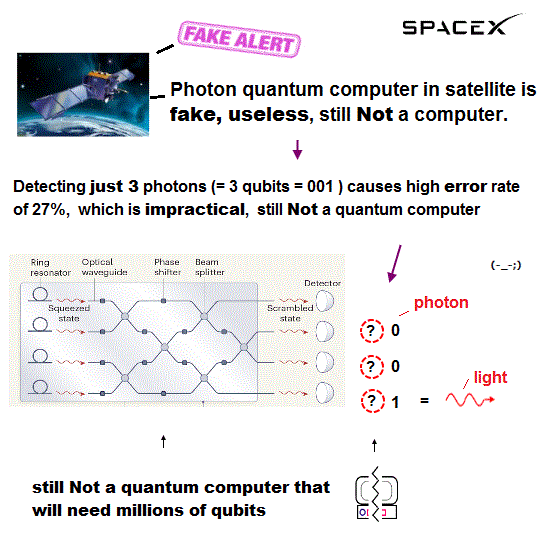As I said, electron spin magnetism ( measured by Stern-Gerach ) is too weak to cause actual stable ferromagnetism.

This is the reason physicsits invented artifcial concpet (= "exchange force" ) in ferromagnet and Pauli exclusion principle, and parameter J is freely chosen, so useless.

Instead of relying on artificial "exchange force", we can naturally explain this stable ferromagnetism using realistic electron's orbital motion.

Each electron orbital motion synchronizes with neighboring ones to generate macroscopic magnet due to Coulomb attraction between electron and nucleus.

Coulomb force is far stronger and more stable than "spin magnetic" interaction, and this orbital motion can explain why iron ferromagnet is stable even at high temperature

## An integer times de Broglie wavelength is needed

### [ to avoid destructive interference of de Broglie wave. ]

(Fig.5)  Quantum orbit satisfies an integer times de Broglie wavelength.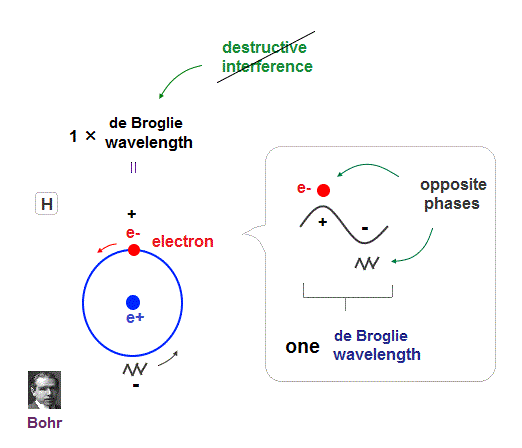Only Coulomb force is insufficient to explain why atomic energy levels are quantized, and why an electron doesn't fall into nucleus.

Bohr model succeeded in getting actual atomic energies, proposing each orbit is an integer times de Broglie wavelength, as well as Schrodinger's hydrogen .

An integer multiple of de Broglie wavelength means an electron can avoid destructive interference and be stable.

Without this de Broglie wave, each electron can be attracted to positive nuclei, until they stick to each other and its energy is unlimitedly lower !

So the repulsive force by electron's de Broglie wave is strong enough to keep the electron away from the nucleus and cause Pauli exclusion force.

## Why Old Bohr model Helium is wrong ?

### [ Old Bohr's Helium causes "destructive" interference. ]

(Fig.6)  Opposite phases of two de Broglie waves cancel each otherIn old Bohr's helium, two electrons are moving on the opposite sides of the nucleus in the same circular orbit (= one de Broglie wavelength ).

Considering Davisson-Germer interference experiment, two electrons of old Bohr's helium are clearly unstable.

1-de Broglie wavelength orbit consists of a pair of the opposite wave phases (= ±ψ ), which cancel each other by destructive interference.

Due to Coulomb repulsion between two electrons, one is always on the opposite side of another where the opposite de Broglie wave phases cancel each other.

Actually, old Bohr's helium of Fig.6 gives wrong ground state energy of helium, when you calculate it.

Old helium gives the total energy of -83.33 eV, which is a little lower than the actual value of -79.005 eV (= 1st + 2nd ionization energy of this ).

## de Broglie wave interference explains helium.

### [ Two de Broglie waves cross perpendicularly = stable. ]

(Fig.7)  Actual Helium must avoid "destructive interference".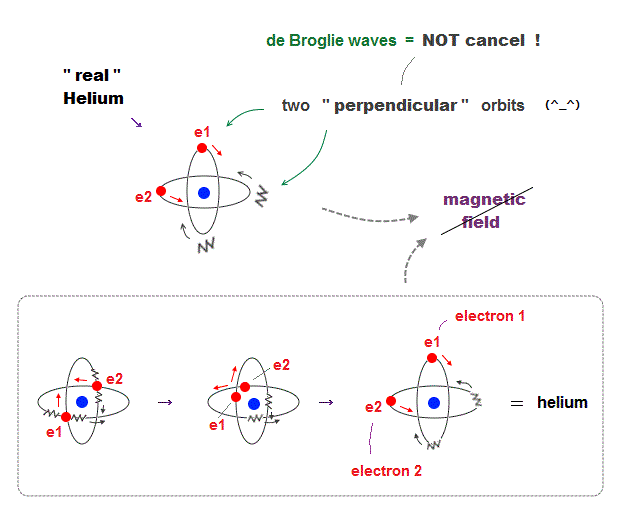If two 1 × de Broglie wavelength orbits are in the same plane in old Bohr's helium model, their opposite wave phases cause destructive interference and vanish.

To avoid vanishing de Broglie's wave, two electron orbits in actual helium must be perpendicular to each other.  Each orbit is one-de Broglie wavelength.

If the two orbits are perpendicular to each other, their wave phases are independent from each other and can be stable, not canceling each other.

This helium model considering actual de Broglie wave interference just agrees with experimental results of all atoms !

## Helium with two closed orbits

### [ Why Hydrogen has magnetic moment, Helium doesn't ? ]

(Fig.8) Hydrogen, Helium with 1 × de Broglie wavelength.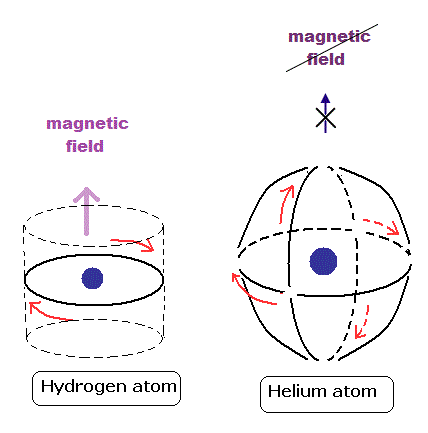These orbits are all just one de Broglie's wavelength.

In this new helium, the two symmetrical orbits crossing perpendicularly are wrapping the whole helium atom completely.

The Bohr model hydrogen which has only one orbit, can not wrap the direction of the magnetic moment completely.

It is just consistent with the fact of the strong stability and the closed shell property of helium.

## Why Helium has NO magnetic field ?

### [ Two opposite de Broglie waves cancel each other in Helium ]

(Fig.9)  Two "opposite" wave phases cancel each magnetic field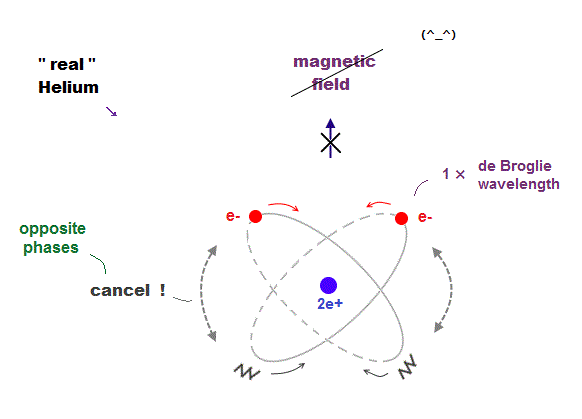One-electron Hydrogen generates magnetic field, while two-electron Helium doesn't.  Why ?  We can explain it using de Broglie waves.

In "actual" Helium model, the opposite phases of two electron's de Broglie waves cross each other perpendicularly.

Because two electrons repel each other by Coulomb repulsion, an electron tends to approch the opposite phase ( where electron doesn't exist ) of another electron.

From a distance (= a third person ), the total magnetic field caused by electron's movement (= de Broglie wave ) is cancelled out to be zero by destructive interference.

So crossing between the opposite de Broglie wave phases is the reason why Helium has NO magnetic field.

## Electron's opposite wave phases cancel each other

### [ Two Helium orbits with opposite phases cancel magnetic field ]

(Fig.10)   ↓ Helium -- two "opposite" wave phases always cross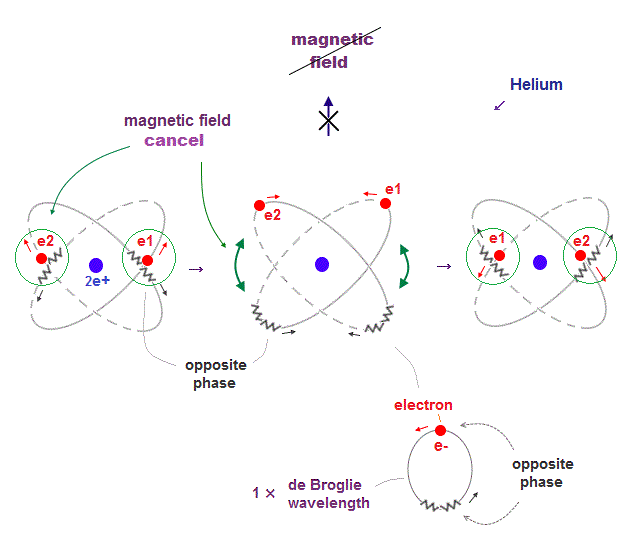When electron's opposite de Broglie wave phases cross each other in Helium two orbits, it can get experimental energy.

Opposite wave phases always cross, so its total magnetic field caused by electron's motion (= de Broglie wave ) is cancelled out to be zero, from a third observer,

## Neon -- 2 × de Broglie wavelength

(Fig.11)  2 × de Broglie wavelength orbits. Each is a pair of opposite phases.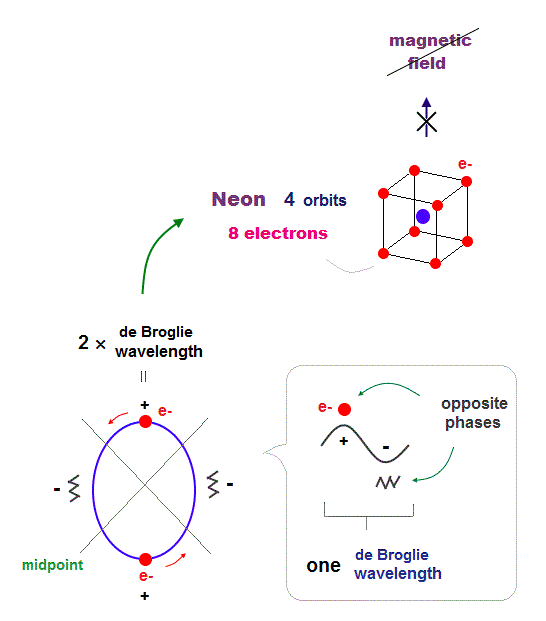One wavelength consists of a pair of "crest" (= + ) and "trough" (= - ) irrespective of transverse and longitudinal waves.

Here we suppose "+" phase contains an electron itself, and "-" phase is compressed by the electron's movement,   --- which is " de Broglie wave ".

2 × ( 1 × ) de Broglie wavelength orbit contains two ( one ) pairs of ±opposite phases and two ( one ) midpoint lines.

These "opposite" wave phases cancel each other by destructive interference.
To avoid it, two orbits must cross perpendicularly in all atoms.

## Difference in 1 and 2 de Broglie wavelengths

### [ 1 × de Broglie wavelength orbits "always" cross each other. ]

(Fig.12)  2 × de Broglie wavelenght orbits contain "free" electrons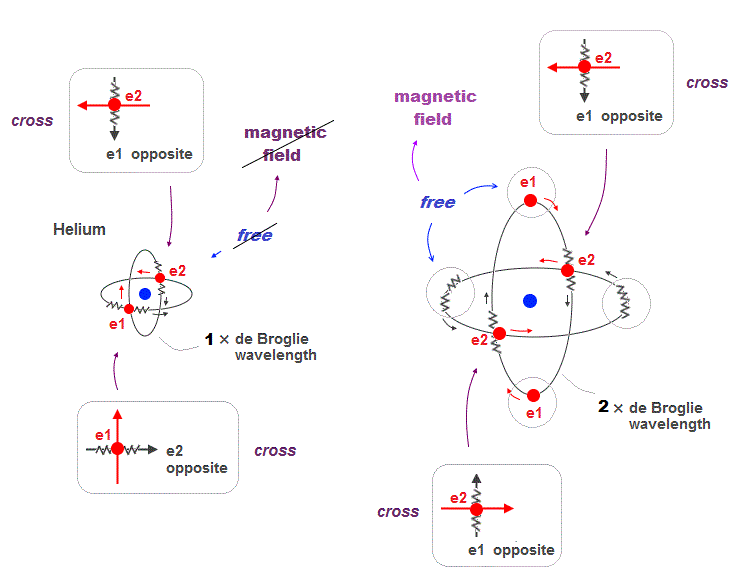Helium two 1 × de Broglie wavelength orbits always cross their opposite (anti) wave phases at right angle.

On the other hand, when two 2 × de Broglie wavelength orbits cross each other, they contain "free electrons" and "free opposite phases" which aren't crossing.

So there is still a room for more orbits in 2 × de Broglie wavelength.

## "Free" de Broglie wave can show magnetic field

### [ Free electrons not crossing opposite phase cause magnetism ]

(Fig.13)  Two 2 × de Broglie wavelength orbits include "free" electrons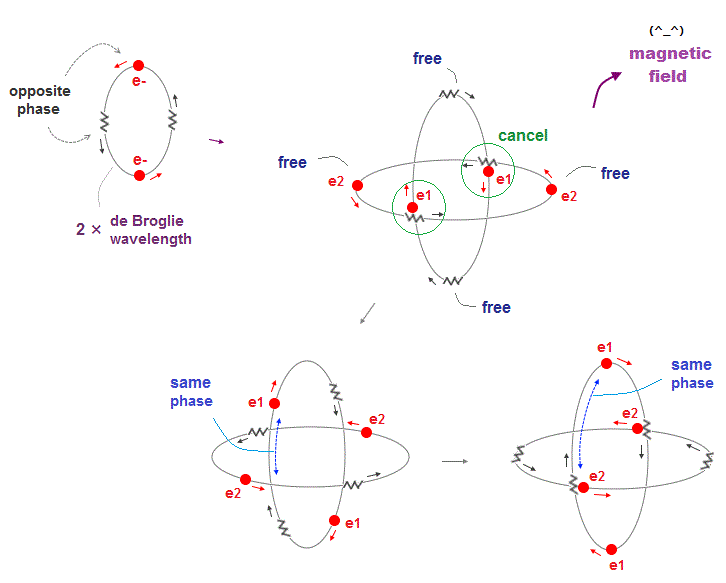Compared to 1 × de Broglie wavelength orbits in Helium, a 2 × de Broglie wavelength orbit includes more different phases.

As one electron moves away from another orbit, its de Broglie wave phase gets free, not cancelled out by its opposite phase.

Magnetic field is caused by electron's motion (= de Broglie wave ), so these "free" electron wave phases can be used as a source of magnetic ferromagnetism.

## 2 × de Broglie wavelength → maximum 4 orbits

### [ Neon consists of 8 electrons in 4 orbits with 2 × wavelength. ]

(Fig.14)  All opposite wave phases just cross each other in Neon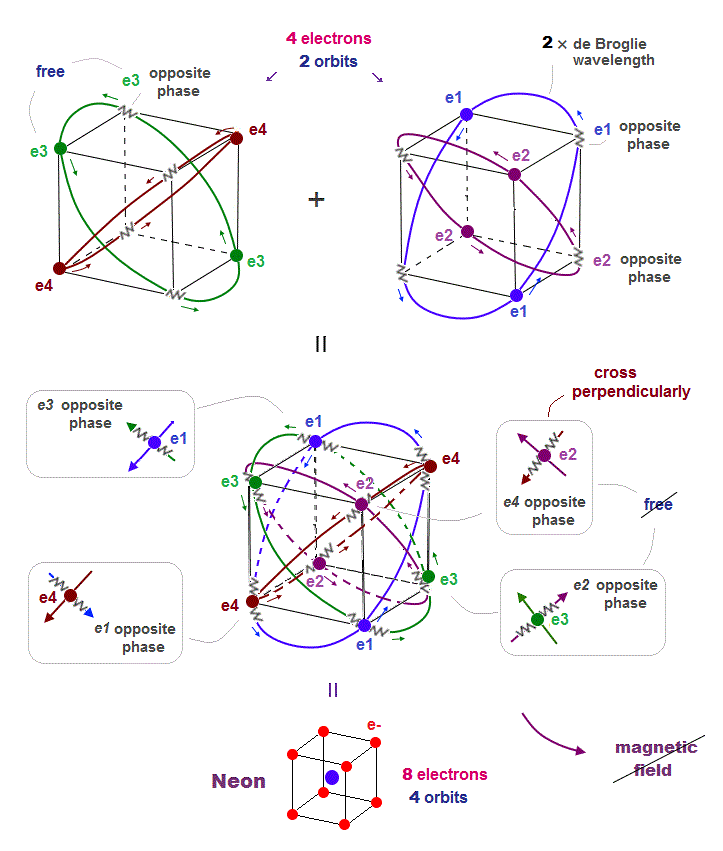Neon contains eight valence electrons.  Neon belongs to the 2nd line in periodic table, meaning its orbit is 2 × de Broglie wavelength.

When just two 2 × de Broglie wavelenght orbits cross each other (= Fig.14 upper ), some electrons are free from their opposite wave phases.

With four orbits, all electrons and their opposite wave phases cross each other, forming stable noble gas Neon with No more room.

The fact that all opposite wave phases always cross means this Neon causes NO magnetic property.

## The periodic motions of eight valence electrons in Neon.

### [ Motion of Neon 8 electrons included in 4 orbits. ]

(Fig.15)  Orbits of Neon cross each other "perpendicularly".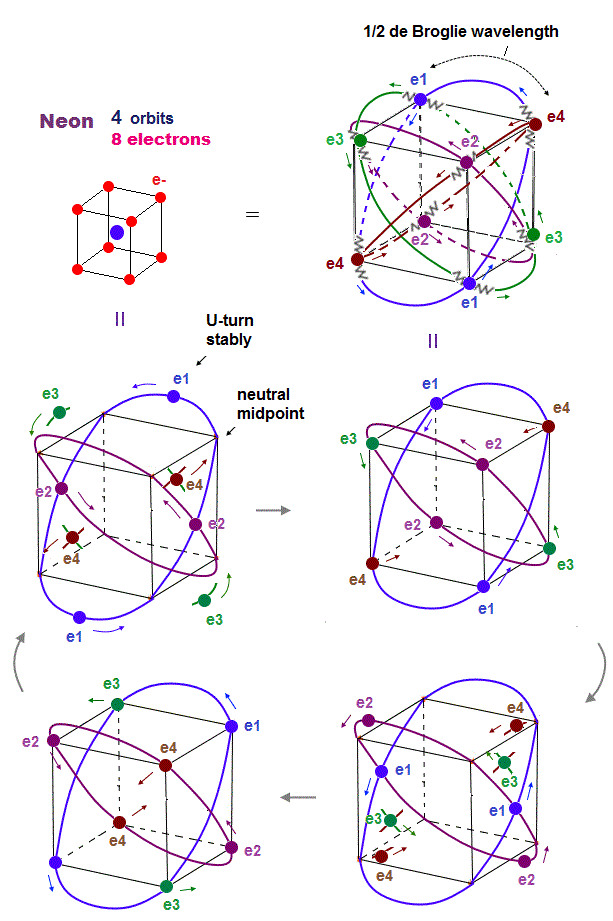As shown on this page, we can show the appropriate new Neon model, in which orbits can cross each other "perpendicularly".

"Perpendicular" crossing means they can avoid "destructive" interference.  Neon consists of four 2 × de Broglie wavelength orbits ( total 4 × 2 = 8 electrons )

## de Broglie "wavelength" determines the maximum orbits.

(Fig.16)  Maximum orbits = midpoint lines + 2 (= two perpendicular orbits )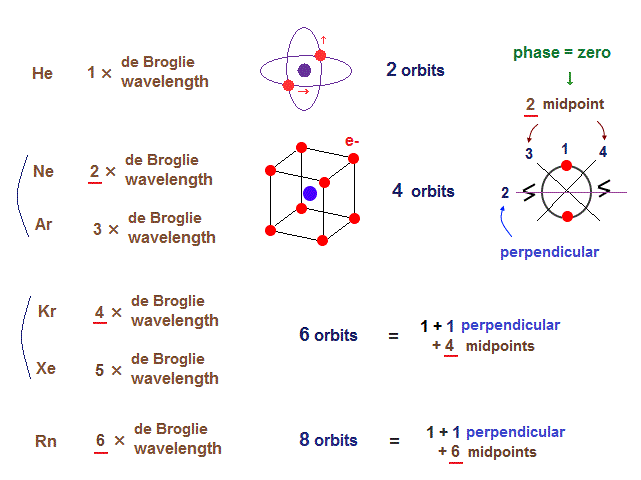As shown on this page, the number of midpoint lines ( related to de Broglie wavelength ) influences the number of maximum orbital number in the periodic table.

The maxium number of orbits in Ne becomes "4" (= 2 × perpendicular + 2 × midlines ).
4 × de Broglie wavelength contains 4 midlines, so the total orbital number of Kr becomes "6".

The odd numbers of "3", "5", "7" orbits are asymmetrical and unstable.
So the orbital numbers of "Ar" (= 3 × waveslength ), "Xe" (= 5 × waveslength ) remain the same as "Ne" and "Kr".

## Six orbits of Krypton valence electrons.

(Fig.17)  6 orbits of Kr outer electrons.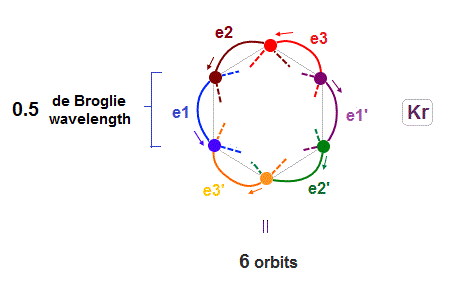Like Neon (= 4 orbits ), Krypton six orbits generate three pairs, each contains two perpendicular orbits with opposite phases and opposite directions ( e1-e1', e2-e2', e3-e3' ).

Fig.17 is Krypton orbits seen from the upper side.
0.5 × de Broglie wavelength orbit is included between two closest intersections, like Neon and Argon.

## Krypton -- all opposite wave phases cross each other

### [ When there are 6 orbits with 4 × de Broglie wavelength, ]

(Fig.18)  All opposite wave phases just cross each other → No magnetic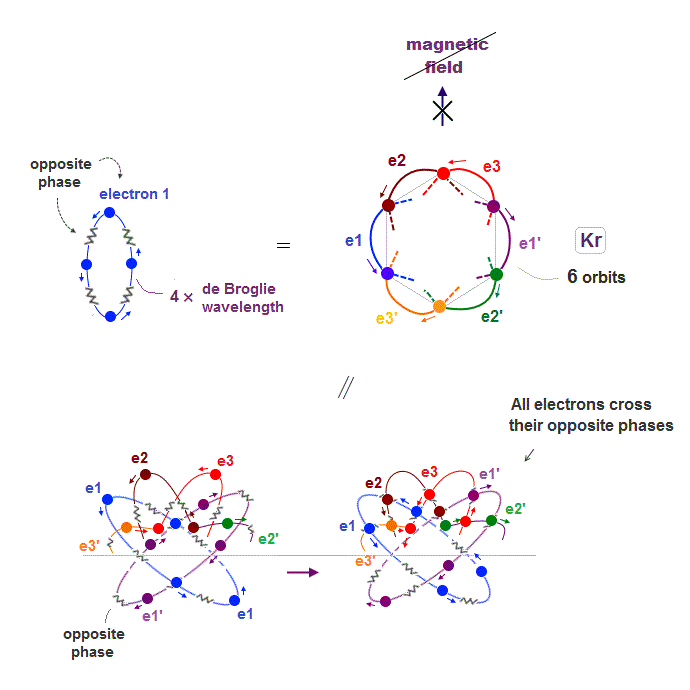Krypton is in the fourth row of periodic table, which means it has 4 × de Broglie wavelength orbit.

If there are 6 orbits with 4 × de Broglie wavelength, all their electron's opposite wave phases always cross each other, and cancel their magnetic property

## Why Krypton has "18" valence electrons ?

### [ To avoid Coulomb repulsion, Krypton decreases electrons to 18 ]

(Fig.19)  When Krypton contains 24 electrons, their repulsion is too strong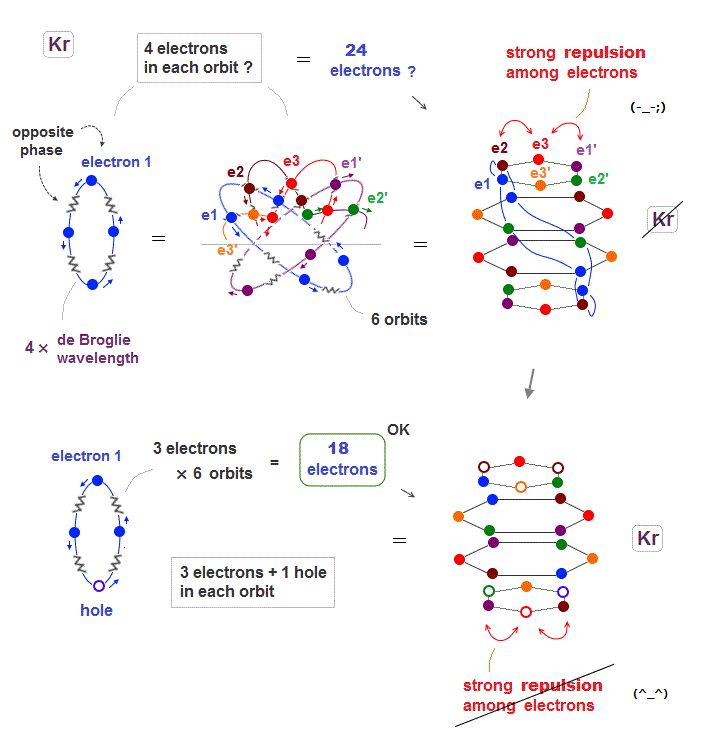Seeing the fourth row in periodic table including 18 elements, we can think noble gas Krypton has the maximum 18 valence electrons.

When Krypton with 4 × de Broglie wavelength orbits has 6 orbits, it can have the maximum 24 electrons (= 4 × 6 ) ?  Unfortunately not.

Kryton orbitals consists of four layers in vertical direction, with each having six electrons, as seen in Fig.19 upper.

But in this case, each six electrons included in the top and bottom layers show stronger Coulomb repulsion than other two middle layers.

So to be symmetric in Coulomb repulsion, the top and bottom orbital layers must decrease electrons in their most stable state ( Fig.19 below ).

Like swing of a pendulum, electrons moving back and forth between unstable and stable states with maximum 18 electrons.

## Ferromagnetic metals -- Fe, Ni, Co

### [ What determines ferromagnetic property in metals ? ]

(Fig.20)  Why only Fe, Co, Ni, Gd-Tm are ferromagnet ?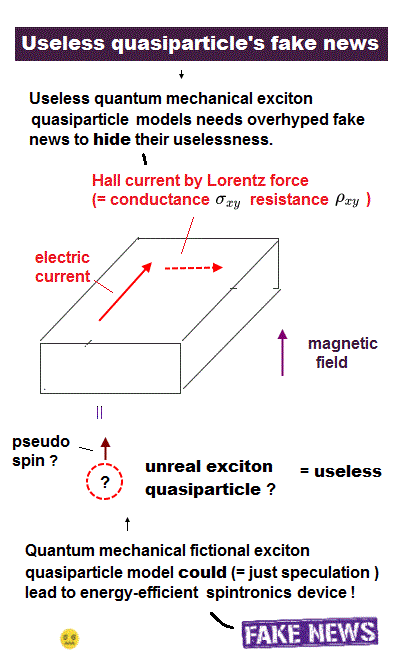There are only a limited number of metals showing ferromagnetism.  Most stable ferromagnetic metals are Fe, Co, Ni in the fourth row of periodic table ( this p.2 )

Besides them, some 4f-rare earths such as Gd, Tb, Dy, Ho, Er, Tm show weak ferromagnetism.

What on earth determines whether each metal is ferromagnet or not ?

## Why metals with few valence electrons cannot be ferromagnet ?

### [ Potassium with one valence electron is always changing its orbit (= magnetic direction ) ! ]

(Fig.21)  Metals with few valence electrons cannot fix magnetic direction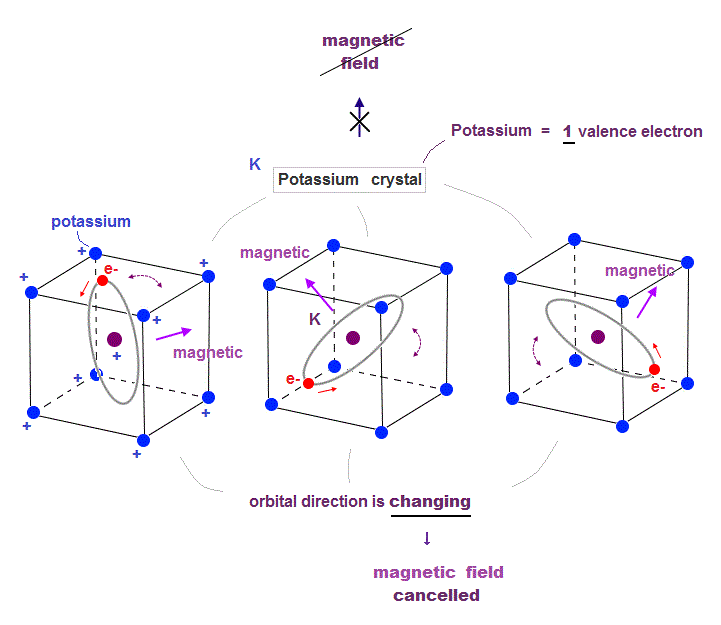In the fourth row of periodic table, Potassium (= K ) has only one valence electron, and its crystal structure is body-centered cubic.

If this potassium has only one orbit, it means there are free electron's de Broglie wave phases which can be used to generate ferromagnetism ?

But metals with a small number of valence electrons cannot be ferromagnet causing stable magnetic field.

Because the electron's orbit of potassium has to move around to keep links to all surrounding electrons, which cannot fix its stable magnetic direction.

To cause stable ferromagnetism, metals must contain electrons' orbits "enough" to fix their magnetic direction.

## Many orbits contained → cancel magnetic field

### [ Krypton having 6 orbits "cancels" all free de Broglie waves for ferromagnetism ]

(Fig.22)  Krypton (= Kr ) has 6 orbits with 4 × de Broglie wavelength → NO magnetic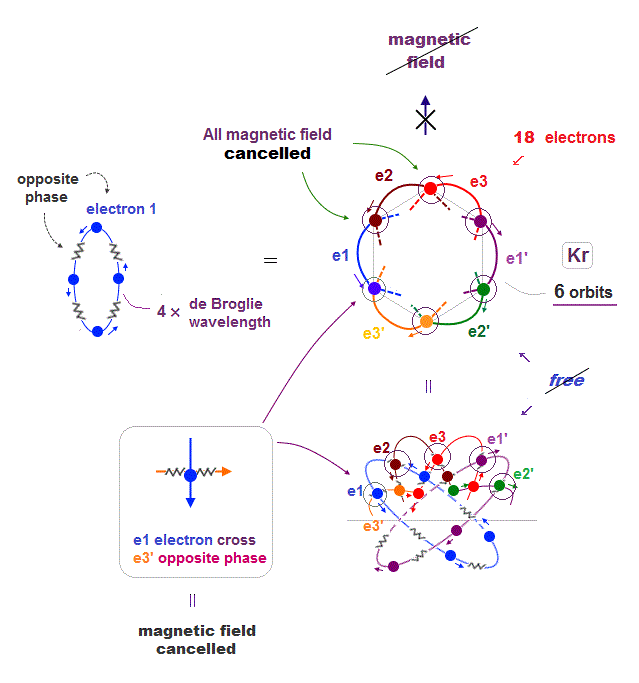If Krypton (= Kr ) has 4 × de Broglie wavelength orbit, it can contain the maximum 6 orbits.

In this state, all electron's "opposite" wave phases just cross each other and cancel their total magnetic field from a distance.

It means if Krypton decreases its orbits (= electrons ), some electrons can be free from other opposite phases, and cause ferromagnetism.

## Why iron (= Fe ) can be stable ferromagnet ?

### [ Fe with 4 orbits has "room" for cause ferromagnetism ]

(Fig.23)  Fe has 8 valence electrons (= 2 × 4 orbits ) → magnetic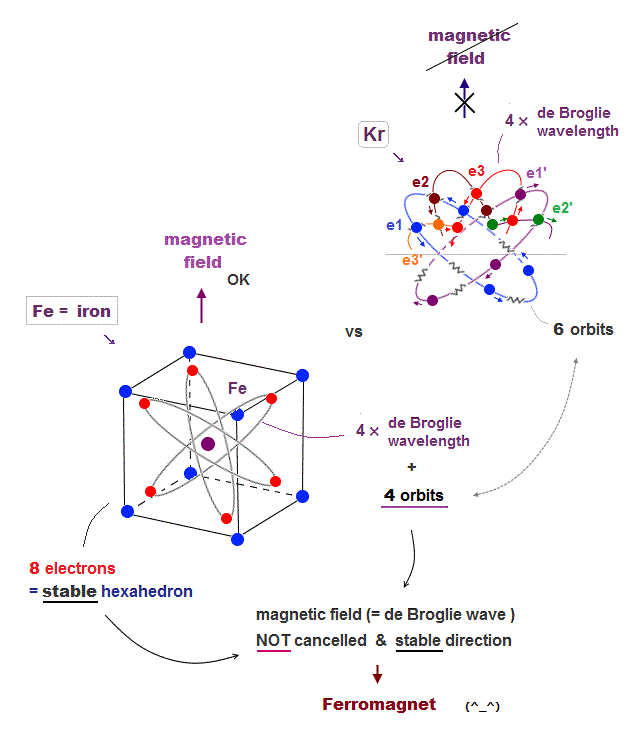Iron (= Fe ) belongs to the 4th row in periodic table, like Kr and K.  It means each orbit of Fe with 8 valence electrons is also 4 × de Broglie wavelength.

We can think Fe has 4 orbits and each orbit include 2 electrons ( 8 = 2 × 4 orbits )

Iron "hexahedral" structure ( with 8 electrons ) can keep its stable links to surrounding electrons and its magnetic direction unlike Potassium.

The fact that Fe contains only 4 orbits ( in 4 × de Broglie wavelength ) means some electrons are free from thier opposite phase, and can cause magnetic field.

## What determines "ferromagnet" ?

### [ Stable magnetic direction & free electrons = ferromagnet ]

(Fig.24)  Why only three Fe, Co, Ni show ferromagnetism ?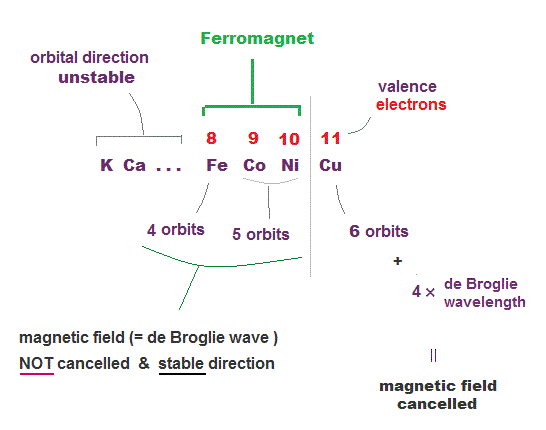To show stable ferromagnetism, metals have to satisfy two important conditions.  One is electron's orbit needs to be "fixed" to cause stable magnetic field.

So matals with a too small number of valence electrons ( like K ) cannot keep its stable magnetic direction, and cannot be ferromagnet.

Second, there must be free electrons left to cause magnetic field.  When orbital number is maximum like Kr, all electrons's de Broglie waves are cancelled by thier opposite phases.

We can think Fe, Co, Ni has 4 ~ 5 orbits in 4 × de Broglie wavelength, which can satisfy these two conditions for ferromagnet.

## 4f-rare earth ferromagnet

### [ 4f-elements has 6 × de Broglie wavelength. -- maximum 8 orbits. ]

(Fig.25)  4f-elements has 5 ~ 7 orbits with "free" electrons.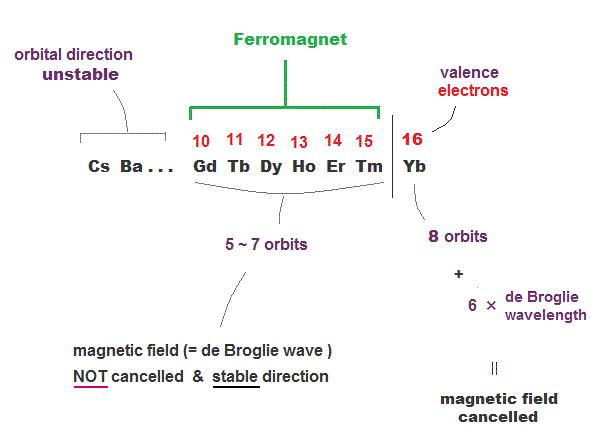4f rare earth metals belong to 6th row of periodic table which means they have 6 × de Broglie wavelength orbits.

Elements with 6 × de Broglie wavelength can have the maximum 8 orbits.  We can estimate Yb has 16 valence electrons (= 2 × 8 orbits ).

So Gd-Tm rare earths have 5 ~ 7 orbits with "free" electrons causing ferromagnet.

6 × de Broglie wavelength orbits have more room than 4 × de Broglie wavelength, so to fix their magnetic direction, more electrons are needed.

So the reason why Gd, Tb, Dy .. Tm are weak ferromagnets is that they also satisfy two important conditions.

Odd de Broglie wavelength orbits (= 5th row in periodic table ) are asymmetric in electrons' positions, so orbital motions are unstable and cancel magnetic property.2016/9/16 updated. Feel free to link to this site.